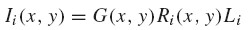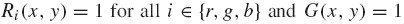# 前言

（1）什么是色彩恒常性？what is Color Constancy？

（2）retinex是什么意思？what is retinex？

retinex 是来自于中古拉丁文Retina 和拉丁文Cortic两个单词的组合， 发音[re-tin-ex] 或 ['ret-nex] 。由Edwin Land 在他的人类色彩视觉模型中提出，是结合眼睛的视网膜(retina)和大脑的头皮层(cortex)而创新出来的一个词。在图像处理中，这个词可以更加明确的定义为一个自动将视觉现实转换到图像的过程，让图像象你实际看见的世界一样的明亮、清晰和清楚。

（原文：from Medieval Latin retina and Latin cortic. Edwin Land coined word for his model of human color vision, combining the retina of the eye and the cerebral cortex of the brain. More specifically defined in image processing as a process that automatically provides visual realism to images.) 来自：Retinex Image processing NASA Langley Research Center

# 灰度世界算法（Gray World Algorithm）

#### 一、灰度世界算法

(1)一般有两种方法确定  :

(2)计算  三个通道的增益系数：  ;  ;  ；

(3)根据Von Kries对角模型，对于图像中的每个像素  ，调整其分量  分量：

#### 二、Matlab实现

``````I=imread('N.jpg');
y=GrayWorld(I);
figure(1);imshow(I);
figure(2);imshow(y);
function y=GrayWorld(Image)
r=Image(:,:,1);
g=Image(:,:,2);
b=Image(:,:,3);
avgR = mean(mean(r));
avgG = mean(mean(g));
avgB = mean(mean(b));
avgRGB = [avgR avgG avgB];
grayValue = (avgR + avgG + avgB)/3
scaleValue = grayValue./avgRGB;
newI(:,:,1) = scaleValue(1) * r;
newI(:,:,2) = scaleValue(2) * g;
newI(:,:,3) = scaleValue(3) * b;
y=newI;``````

（0）图像成像模型

White Patch Retinex算法的目的是恢复图像的本身色彩，这势必就要估计图像中外界光源的强度。下面是图像成像的数学表达式：（2）

（1）基本算法

（2）改进算法

### White Patch Retinex

（1）图像成像模型

（2）最原始的White Patch Retinex算法

White Patch Retinex算法的主要思想是：if there is a white patch in the scene, then this patch reflects the maximum light possible for each band.This will be the color of the illuminant.举例说明，如果（3）改进后的White Patch Retinex算法MATLAB代码：

1. function out = WhitePatchRetinex(in,para)
3. if( nargin < 2 )
4. para = 0;
5. end
6. out = zeros(size(in));
7. inDouble = double(in);
8. L = [0 0 0];
9. if ( para == 0 )
10. for i = 1:3
11. L(i) = max(max(in(:,:,i)));
12. out(:,:,i) = inDouble(:,:,i)/L(i);
13. end
14. elseif ( para == 1 )% more robust algorithm
15. np = 0.1 * size(in,1)*size(in,2);
16. for i = 1:3
17. H = imhist(in(:,:,i));
18. j = 256;
19. sum = 0;
20. while( (sum < np)&(j > 1) )
21. j = j-1;
22. sum = sum + H(j);
23. end
24. L(i) = j;
25. out(:,:,i) = inDouble(:,:,i)/L(i);
26. end
27. end

• 发表于 2020-03-01 23:17:36
• 阅读 ( 1910 )
• 分类：Go